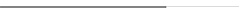## 2021年成人高考高起点文史财经类数学考试真题及答案

1.设集合A={0,1}，B={0,1,2}，则

A.{1，2}   B.{0，2}   C.{0，1}   D.{0，1，2}

2.函数y=2sinxcosx的最小正周期是

A.π/2  B.4π  C.2π  D.π

3.等差数列{an}中，若a1=2，a3=6，则a7=

A.10   B.12   C.14    D.8

4.若甲：x＞1；乙：ex＞1，则

A.甲是乙的必要条件，但不是乙的充分条件

B.甲是乙的充分必要条件

C.甲不是乙的充分条件，也不是乙的必要条件

D.甲是乙的充分条件，但不是乙的必要条件

5.不等式|2x-3|≤1的解集为

A.{x|1≤x≤2}   B.{x|x≤-1或x≥2}

C.{x|1≤x≤3}   D.{x|2≤x≤3}

6.下列函数中，为偶函数的是

A.y=log2x

B.y=x2

C.y=4/x

D.y=x2+x

7.点（2,4）关于直线y=x的对称点坐标为

A.（4,2）   B.（-2,-4）   D.(-2,4)   D.(-4，-2）

8.将一颗骰子抛掷1次，得到的点数为偶数的概率为

A.2/3   B.1/6   C.1/3   D.1/2

9.在三角形ABC中，若AB=3，A=45°，C=30°，则BC=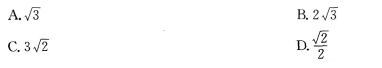10.下列函数中，函数值恒为负值的是

A.y=x   B.y=-x2-1   C.y=x3   D.y=-x2+1

11.过点（0,1）且与直线x+y+1=0垂直的直线方程为

A.y=x+1   B.y=2x+1   C.y=x   D.y=x-1

12.设双曲线x2/16-y2/9=1的渐近线的斜率为k，则|k|=

A.9/16   B.16/9   C.4/3   D.3/4

13.642/3+log1/381=

A.8   B.14   C.12   D.10

14.若tanα=3,则tan(α+π/4)=

A.-2   B.1/2   C.2   D.-4

15.函数y=ln(x-1)2+1/(x-1)的定义域为

A.{x|x＜-1或x＞1}

B.{x|x＜或x＞1}

C.{x|-1＜x＜1}

D.R

16.某同学每次投篮投中的概率，该同学投篮2次，只投中1次的概率为

A.12/25  B.9/25   C.6/25   D.3/5

17.曲线y=x3-4x+2在点（1，-1）处的切线方程为

A.x-y-2=0

B.x-y=0

C.x+y=0

D.x+y-2=0

18.若平面向量a=(x,1),b=(1,-2),且a//b，则x=

19.若二次函数f(x)=ax2+2x的最小值为-1/3，则a=

20.某次测试中5位同学的成绩分别为79,81,85,75,80，则他们的成绩的平均数为

21.函数y=2x-2的图像与坐标轴的交点共有

22.（本小题满分12分）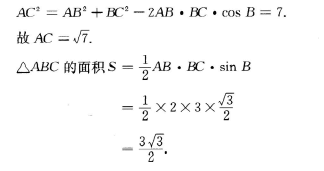23.（本小题满分12分）

（1）求{an}的通项公式；

（2）求{an}的前5项和。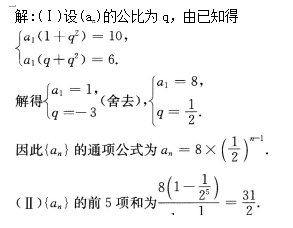24.（本小题满分12分）

（1）求m；

（2）求f(x)的单调区间。

（2）由（1）得，f/(x)=6x2+6x-36,

25.（本小题满分12分）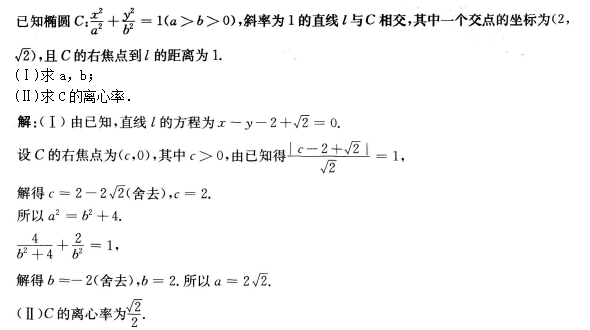## 在线课堂

### 华侨大学夜大学成人高考在线课堂### 华侨大学夜大学自学考试、网络教育在线课堂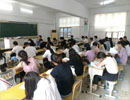### 2022年福建省职称（资格）考试在线课堂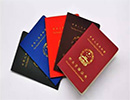2022年福建省职称（资格）考试在线课堂 [详细]